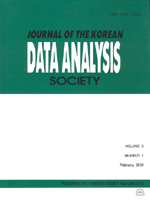상세검색
최근 검색어 전체 삭제
다국어입력
즐겨찾기0KCI등재 학술저널

# Interpreting PLSR and PCR Solutions via Moore-Penrose Generalized Inverse

• 등재여부 : KCI등재
• 2003.06
• 199 - 210 (12 pages)

This paper interprets partial least squares regression (PLSR) and principal component regression (PCR) solutions and data transformations in terms of Moore-Penrose generalized inverse. By finding a Moore-Penrose inverse of the matrix X &#8314; for the solution b = X &#8314; y in a rather backward way, matrix expressions for the transformed X matrices are provided and the way they alter the original X data is shown for the PLSR and PCR methods. A numerical example is given to illustrate how the transformation matrices and the transformed X matrices change as the number of components varies.

1. Introduction

2. PLSR and PCR Solutions in terms of Generalized Inverse

3. Example

4. Summary

References